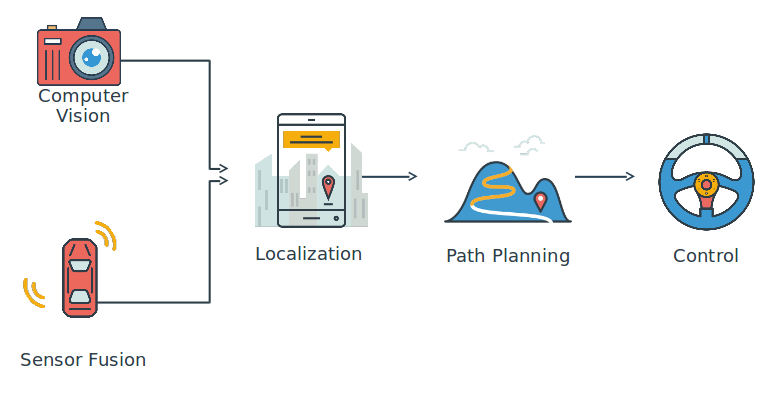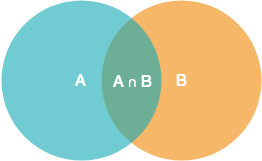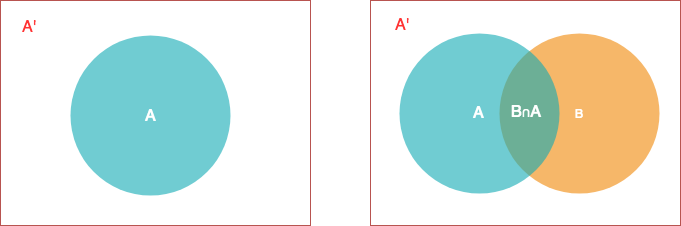# 贝叶斯模型Course Notes from Udacity Self-Driving Car Nanodegree Program

### 条件概率公式1. $P(A) \thinspace = N \thinspace / \thinspace 样本空间$
2. $P(A∩B) \thinspace = M \thinspace / \thinspace 样本空间$
3. $P(B|A) \thinspace = M/N = P(A∩B)/P(A)$

1. 任何时候行驶，有20%的车辆行驶在最左侧车道上
2. 高速上40%的车都是高速行驶的车
3. 对于行驶在最左车道上的车，有90%的车是高速行驶的

### 全概率公式1. 1%的人会的癌症
2. 在得癌症的人中，90%的癌症检测结果都是阳性
3. 在没有得癌症的人中，10%的人检测结果是假阳性（意思是这些人没有得癌症，却被误诊为癌症）

### 贝叶斯定理1. $P(R|A)=0.9$ , 在A区域看到红色的概率为0.9
2. $P(G|B)=0.9$ , 在B区域看到绿色的概率为0.9
3. $P(G|C)=0.9$ , 在C区域看到绿色的概率为0.9

1. $P(A∩R) \thinspace = \thinspace P(R|A) \times P(A) \thinspace = \thinspace 0.9 \times 0.3 \thinspace = \thinspace 0.3$
2. $P(B∩R) \thinspace = \thinspace P(R|B) \times P(A) \thinspace = \thinspace (1-0.9) \times 0.3 \thinspace = \thinspace 0.033$
3. $P(C∩R) \thinspace = \thinspace P(R|A) \times P(A) \thinspace = \thinspace (1-0.9) \times 0.3 \thinspace = \thinspace 0.033$

1. $P(A|R) \thinspace = \thinspace \frac {P(R∩A)}{P(R)} \thinspace = \thinspace \frac{0.3}{0.56} \thinspace = \thinspace 0.818$
2. $P(B|R) \thinspace = \thinspace \frac {P(B∩A)}{P(R)} \thinspace = \thinspace \frac{0.033}{0.56} \thinspace = \thinspace 0.091$
3. $P(C|R) \thinspace = \thinspace \frac {P(C∩A)}{P(R)} \thinspace = \thinspace \frac{0.033}{0.56} \thinspace = \thinspace 0.091$

1. 得到某件事情发生的先验概率 $P(H)$，进而的到 $P(H’)$
2. 根据已有的先验信息，进行某种试验$T$，观察试验结果，得到条件概率： $P(T|H)$ 以及 $P(T|H’)$ , 进而得到 $P(T’|H) = 1-P(T|H)$
3. 计算先验概率和试验概率的联合概率：
• $P(T,H) = P(T|H)*P(H)$
• $P(T,H’) = P(T|H’)*P(H’)$
4. 计算试验结果出现的概率，即全概率: $P(T) = P(T,H) + P(T,H’)$
5. 计算贝叶斯概率，即通过试验矫正后的概率：$P(H|T) = \frac {P(T|H)*P(H)}{P(T)}$

### 自动驾驶中的贝叶斯模型

1. Carmera，采集图像
2. Lidar，360度激光测距

1. 车正在上坡
2. 车左边有一棵树
3. 车轮指向右边

（全文完）

## 附录

### Solution code for project Joy Ride:

car_parameters = {"throttle": 0, "steer": 0, "brake": 0}

def control(pos_x, pos_y, time, velocity):
""" Controls the simulated car"""
global car_parameters
if(time < 3):
car_parameters["throttle"] = -1.0
car_parameters["steer"] = 1
car_parameters["brake"] = 0
elif(pos_y > 32):
car_parameters["throttle"] = -1.0
car_parameters["steer"] = -1
car_parameters["brake"] = 0
else:
car_parameters["throttle"] = 0
car_parameters["steer"] = 0
car_parameters["brake"] = 1

return car_parameters

import src.simulate as sim
sim.run(control)